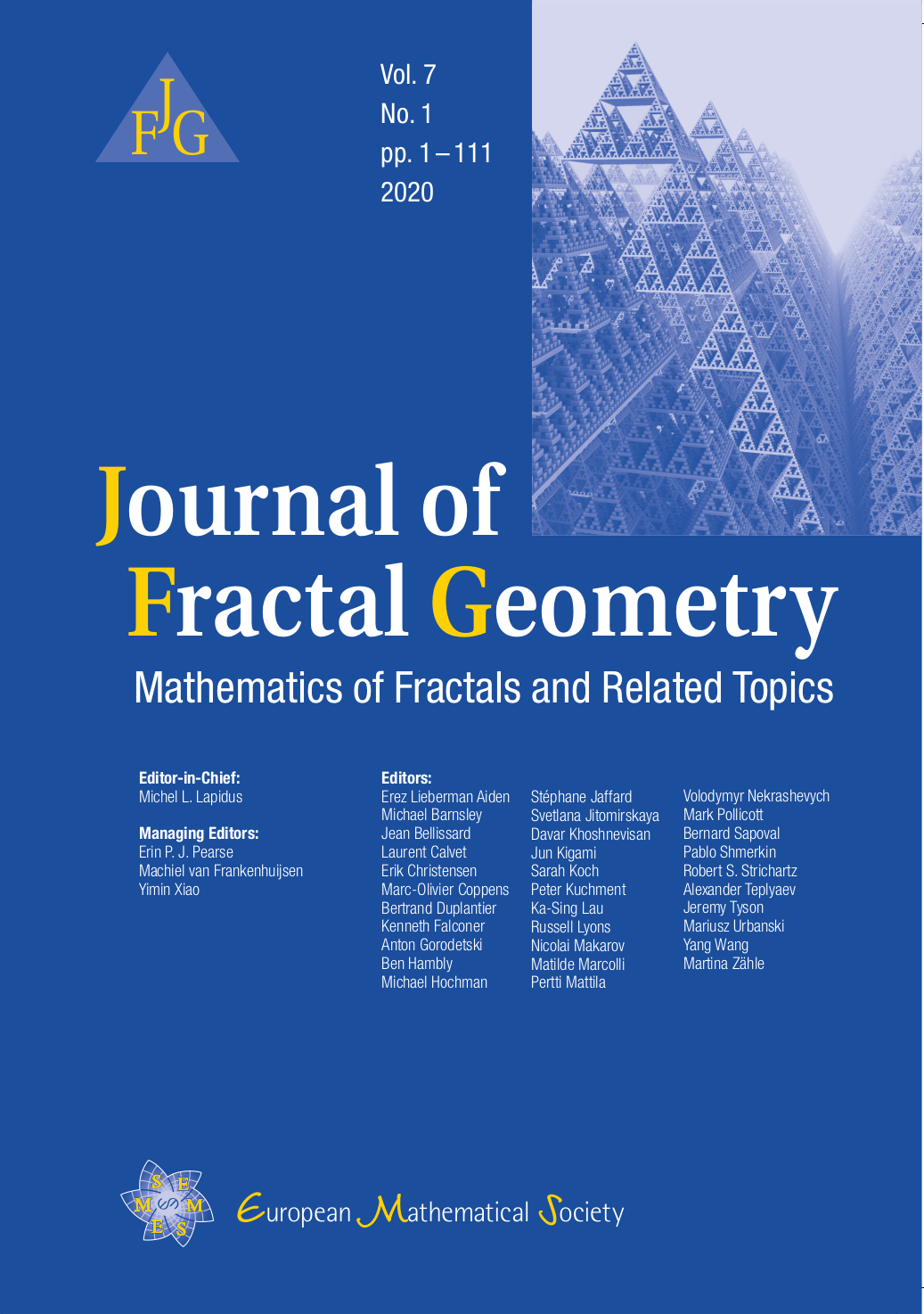JFG

# Journal of Fractal Geometry

DOI: 10.4171/JFG | ISSN: 2308-1309 | eISSN: 2308-1317The Journal of Fractal Geometry is dedicated to publishing high quality contributions to fractal geometry and related subjects, or to mathematics in areas where fractal properties play an important role. Such topics include:

• Self-similarity (broadly interpreted), iterated functions systems

• Analysis and partial differential equations on fractal domains and domains with fractal boundaries

• Multifractality

• Harmonic analysis, including wavelet theory

• Spectral and fractal geometry, direct and inverse problems, fractal spectra

• Dynamical systems, including complex dynamics and symbolic dynamics

• Probability theory and stochastic processes, including SLE and percolation theory

• Number theory and fractal geometry, including zeta functions and Diophantine approximation

• Geometric measure theory and potential theory

• Metric measure spaces, including nonsmooth differential calculus

• Graph theory and other aspects of discrete mathematics, metric and quantum graphs

• Operator algebras and noncommutative fractal geometry

• Self-similar groups and finite automata, quasicrystals, nonarchimedean analysis

• Limit sets of Fuchsian and Kleinian groups, Apollonian packings

• Experimental mathematics and numerical approximations

• Applications to physics, computer science, life sciences, geophysics, economics/finance, and other sciences

The Journal of Fractal Geometry accepts submissions containing original research articles and short communications. Occasionally research expository or survey articles will also be published. Only contributions representing substantial advances in the field will be considered for publication. Surveys and expository papers, as well as papers dealing with the applications to other sciences or with experimental mathematics, may be considered, especially when they contain significant mathematical content or value and suggest interesting new research directions through conjectures or the discussion of open problems.

The Journal of Fractal Geometry is covered in: Mathematical Reviews (MR), Current Mathematical Publications (CMP), MathSciNet, Zentralblatt für Mathematik, Zentralblatt MATH Database, Emerging Sources Citation Index.

This journal adheres to the EMS Code of Practice.